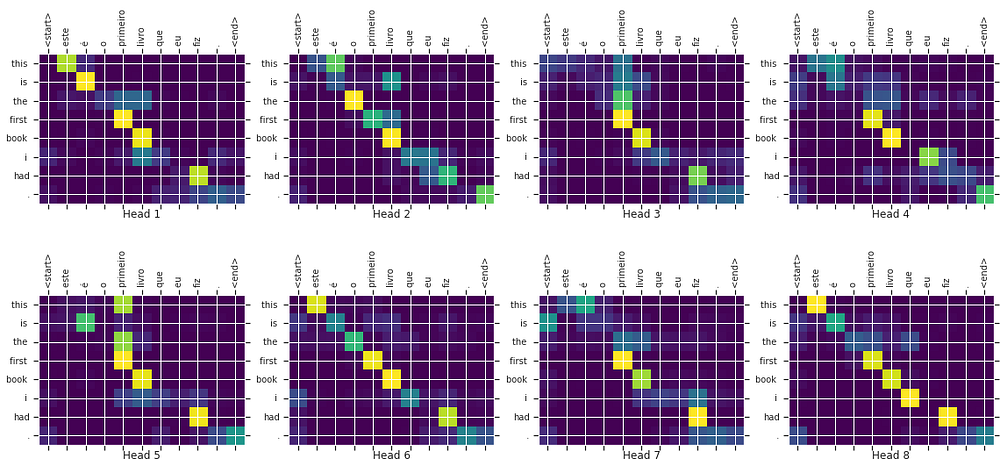# The Mighty Attentions, Optimized

Original article was published on Artificial Intelligence on Medium# The Mighty Attentions, Optimized

Attention is the mightiest layer so far, which symbolizes its parent paper that “Attention is all you need” in true sense. Almost all tasks, be it images, voice, text, reasoning, etc, uses attentions now.

But the layer is very heavy with most of STOA tasks taking days to train. Do we really need so many parameters to learn what’s the intuition behind the Attentions?

We discuss this below.

# Attention As Described.

Attention is described in its true form as

while it is implemented as

Now if we consider Q, K, V coming from the same matrix of size m x n of seq length m and embedding dimension n. And we need to assume a dimension of attention projection lets say that is l.

Now the dimension of the multiplication is as follows

Matrix multiplication for attention then now is

which simplifies to

this totals to 3(n x l) weights. 2 times for the softmax part. Just to estimate an m x m matrix. Which is basically seq_len x seq_len. We don’t have to multiply or need so many weights.

# Direct Attention.

I propose why not have a m x m matrix directly to estimate the, which is similar to a feedforward matrix just with softmax. Softmax forms the key attention part here to stress on certain inputs.

A seq_len x seq_len matrix symbolizing the importance of certain words, in case of machine translation. After all, this is the intuition behind the attentions.

Proposed Direct Attention

Pros

1. Lesser Weights and Faster training.
2. Usefull when attention is input independent or strictly position dependent for example x(t+1) = k0*x(t) + k1*(x(t-1) + k2*x(t-2), auto regressive case.

Cons

1. It only works when attention is independent of the input.
2. Not always useful. except for cases when rearranging the input is required.

# Input Dependent Direct Attention.

Let’s change the order of the matrix calculus and keep the same weights but make it function of the input.

Here the matrix multiplication(*) we do is element-wise. This is constrained on weights and still input dependent. This constraint the learning power of the model as we are using far fewer weights than in the original version. But do we always need so many weights? And after all, we have multi-heads to learn alternative representations.

Pros

1. Lesser Weights and Faster training.
2. Even useful for input dependent attention like language

Cons

1. The assumption here is that m << n if m is comparable to n then m x m weight matrix can be huge. And we may have more weights than original attention.
2. Assumption multi-heads will take care of alternate attentions required.

# Conclusion

I am here not proposing that original attention is not required, instead just that it is not always required in that form. Also, language models can be trained as a mix of these, instead of heavy attention only.